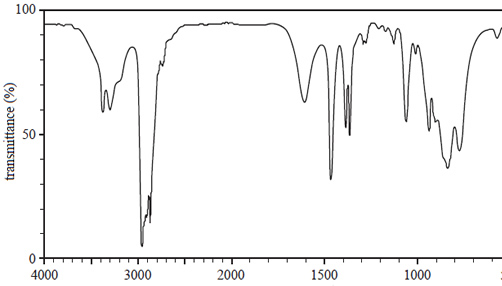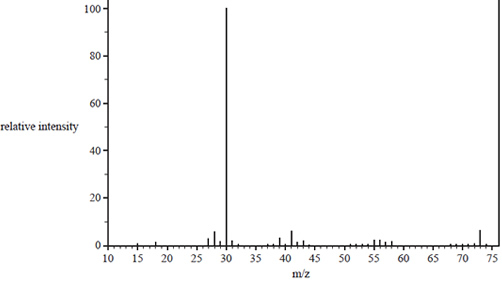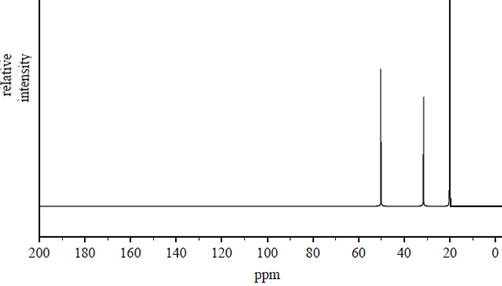Spectroscopy (2018 VCE) 1) A chemical that contains carbon, C, nitrogen, N, and hydrogen, H, in the ratio 4:1:11 is analysed using spectroscopy. a. The infra-red (IR) spectrum of the chemical is shown below.Write the bond responsible for the wave numbers given at wave number Bond 2956 (cm–1) ______________________ 3376 (cm–1) ______________________ Solutionb) The mass spectrum of the compound is shown belowi. What is the molecular formula for the parent molecule? Justify your answer using information from the mass spectrum. ii. Identify the fragment that produces the base peak. iii. Draw the structural formulas for two possible structural isomers of the chemical, which are consistent with the mass spectrum and the IR spectrum. Solutionc) Using the 13C NMR spectrum identify the type of carbons that from peaks at. Chemical shifts 20.0 and 50.2Solutiond) Draw the skeletal formula for the chemical, which is consistent with the IR spectrum, mass spectrum and 13C NMR spectrum.       Solution